# Mach-Zender-Interferometer with polarizers

• I
Sonderval
TL;DR Summary
How do different polarizers added in a MZI change the interference pattern?
I have a question on how exactly polarizing filters would influence interference in a Mach-Zehnder interferometer.
To explain, I'll show some configurations and what I would expect to happen - please tell me if I am incorrect anywhere.

Here is the standard MZI configuration with no filters and a single-photon source on the lower left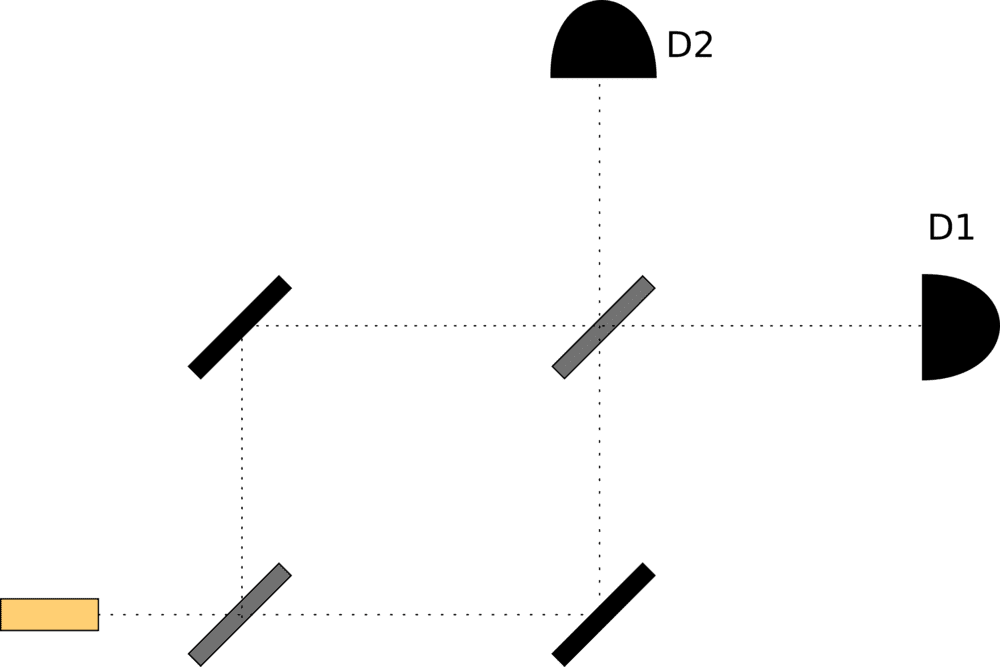In this configuration, 100% of photons will reach D1, none will reach D2, due to the differential phase shifts from the beam splitter. (I assume that both beam splitters are symmetric and shift transmitted and reflected by 90°, as explained here: https://www.physicsforums.com/threa...h-zehnder-interferometer.972867/#post-6188472)
Now I add two polarizing filters, one vertical, one horizontal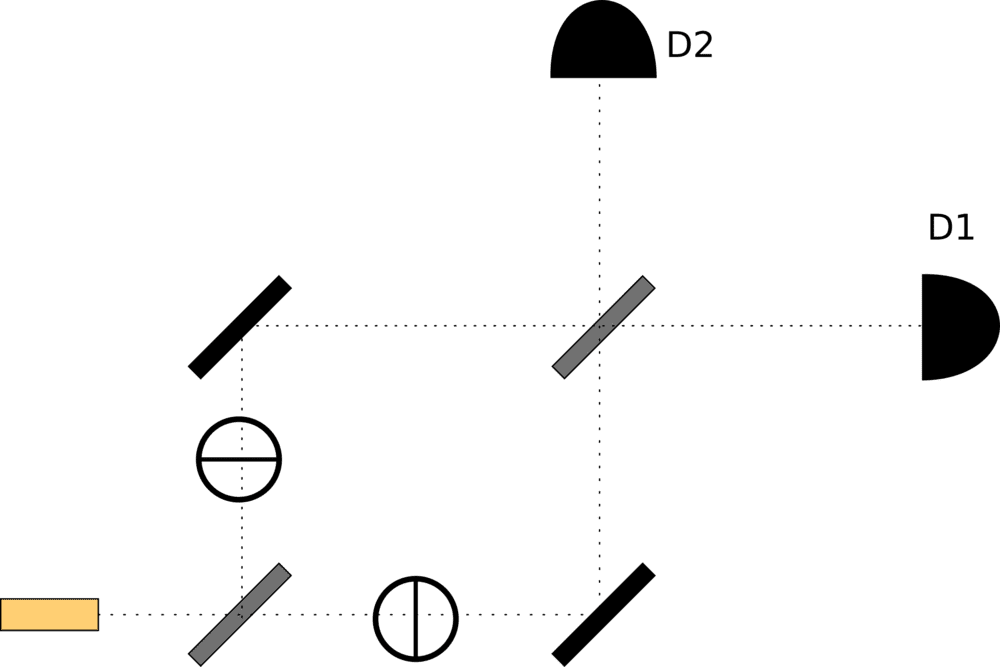In this configuration (with single photons emitted from the light source on the lower left), on average, only every second photon will hit one of the detectors (for simplicity, assume that the light source emits photons with polarization 45°, i.e. a superposition og horizontal and vertical). Of these 50%reach D1, 50% reach D2 because the polarization adds a "which-path information" to the photon state and thus destroys the interference pattern. So overall I have 25% at D1, 25% at D2, 50% lost.

When I now add a third filter at 45° in the D1-path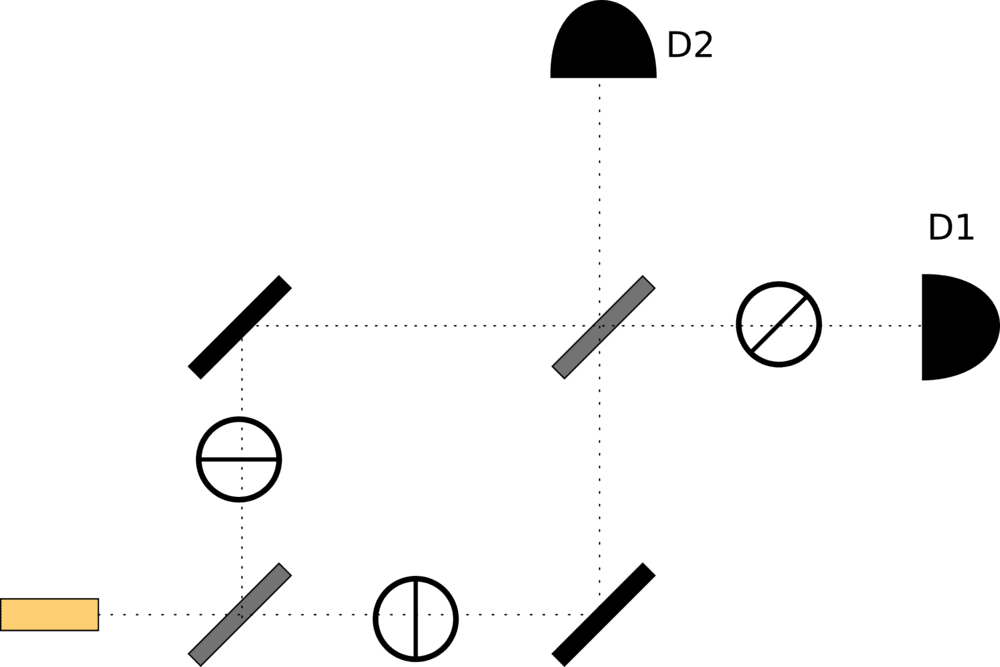I expect that this destroys the which-path information. However, the which-path information is still available at D2, so I would expect now that I have 25% of the photons at D2, 12,5% at D1 (number is halved due to the loss at the third filter).

And finally, I add a filter to the D2-path: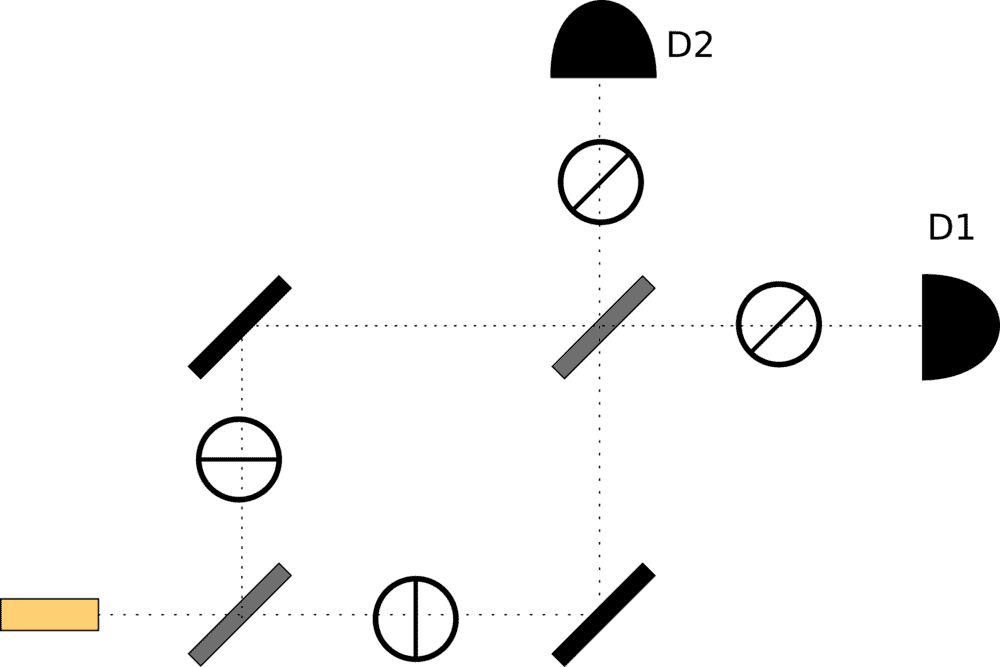Now I've destroyed the which-path information on both paths, so that interference should work again. In this situation I would expect 25% of the photons to end up at D1, none ending up at D2 and the rest being absorbed at one of the filters.

Are all these considerations correct or did I make a mistake somewhere?

Last edited:

charters
Are you saying Alice at D1 expects her average click rate to double based on Bob at D2's choice to place the polarizer in a region spacelike separated from her? Is this not a causality violation?

•Sonderval
Sonderval
@charters
Yupp, that's worrying me as well.

Gold Member
2022 Award
I've not checked it by a full-fledged calculation, but just adding the relative phase shifts naively I think your presentation is correct.

There's no causality violation here. You can come to that conclusion only if you wrongly think about the photons as localized billiard-ball like bullets. This picture is utterly wrong! Rather think about photons as electromagnetic waves of the lowest possible intensity for the given frequency. Then it's clear that you need to consider the entire setup, because the interference at a point is caused by recombining parts of the waves going along different paths, collecting their phase changes while travelling, at one point.

This is of course not a correct picture either if you work with single-photon states since a photon cannot be "devided" in different wave parts, but this refers to the detection of the photons in a detector, and the waves do not describe the photons themselves but only the probabilities for detection events of a photon at the place of the detector (note that photons do not even have a position observable, i.e., it doesn't make sense to talk about the position of the photon; only the detection events have a position given by the position of the detector).

•Nugatory and Sonderval
Sonderval
@vanhees71
I now tried to calculate things explicitly and believe my idea above to be incorrect. (Calculation follows below, I'd be very grateful for someone to check it.)
However, I do see a problem with causality if my idea were correct:
Image we set up the light source to send one photon per second. In scenario 3, Alice at D1 would then expect to find 12.5% of them, so on average one every 8 seconds. When Bob inserts a filter in front of D2, Alice would then find an increased probability of 1/4. With sufficient distance between D1 and D2, I think that this would actually allow to send a message, wouldn't it?

But I think my calculation is incorrect anyway because I did not account for the correct superposition of horizontal and vertical states on the path to D1. I use the following labels for the paths: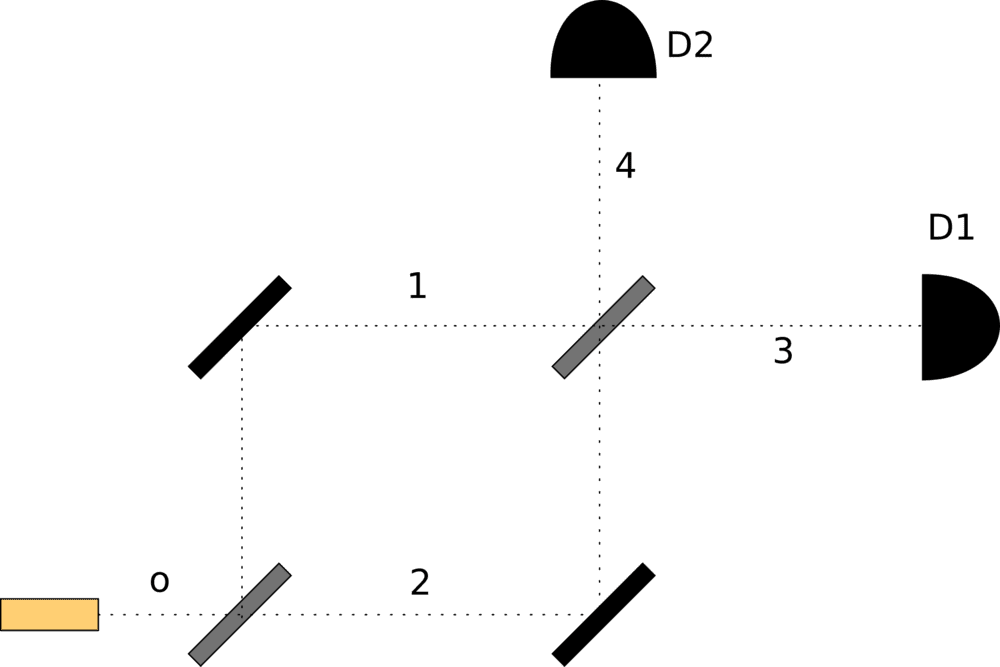The first beam splitter creates
$$|0\rangle \rightarrow \frac{1}{\sqrt{2}} (i|1\rangle + |2\rangle)$$
(as I said, I assume a symmetric BS with 90° phase shift)
The second beam splitter then changes this to
$$\rightarrow \frac{1}{2} (i (i|4\rangle + | 3\rangle) + |4\rangle + i|3\rangle)$$
so the two ##|4\rangle##-terms cancel each other and the full signal ends up in D1.

Adding the polarization state, the final state can be written as
$$\rightarrow \frac{1}{2} (i (i|4 H\rangle + | 3 H\rangle) + |4 V \rangle + i|3 V\rangle)$$
and with this all four terms are orthogonal to each other and I get 50% probability for D1 (25% with H, 25% with V) and the same for D2.

So this seems to be correct (i hope!).

I write the 45-degree polarized state as $$|45\rangle = \frac{1}{\sqrt{2}} (|V\rangle + |H\rangle)$$ or
$$|V\rangle = \frac{1}{\sqrt{2}} (|+45\rangle + |-45\rangle)$$
$$|H\rangle = \frac{1}{\sqrt{2}} (|+45\rangle - |-45\rangle)$$

I can now just write the V- and H- states in the 3-path and get

$$\rightarrow \frac{1}{2} (-|4 H\rangle + |4 V \rangle + \frac{i}{\sqrt{2}} (| 3, +45\rangle - | 3, -45\rangle ) + \frac{i}{\sqrt{2}} (| 3, +45\rangle + | 3, -45\rangle ) )$$

With this, what i now see is that the two states in path 3 actually combine to form a +45°-polarized wave, so no photon should be absorbed at the filter at +45°. Corresponsingly, if I have a +45°-filter on path 4, it will absorb all photons whereas a -45° filter will absorb none.

So the correct probabilities should be that in my situation 3 above, the overall probability at D1 is still 25% since the photons from the two paths actually linearly combine to a +45°-polarization state.

I'm not totally sure if this is the right way to calculate things, though, so if you (or someone else) could check this, I'd be very grateful.

Gold Member
2022 Award
I assumed that the beam splitters don't change the polarization. If you have polarizing beam splitters, of course, the results change. To see this one would have to write down the complete transfer matrices for each of the setup.

Nevertheless, there's no FTL communication possible. Say the message is encoded as "0" and "1", encoded by Bob as having no polarizer (0) vs. having a polarizer (1) in front of his detector, D2. All Alice can measure is however the probability for her detector to click. She needs many photons to get the probability and this takes time. The signals do not propagate faster than light to A and B. Also for the setup to work the photons need to be sufficiently coherent, i.e., they should have a pretty well determined energy. That means the uncertainty of their emission time gets pretty large (through the energy-time uncertainty relation). In order to be able to get enough statistics the photons need to come however well separated in time, i.e., you have to wait sufficiently long between sending the photons, and, because the time uncertainty of the single photons is pretty large, this time is pretty long.

Sonderval
@vanhees71
I'm not sure I understand. The beam splitters do not change polarization here, polarization comes into it only in situation 3 and 4 where I explicitly added the filters. That is why i simply added the appropriate H and V to the states in the 3rd equation.
I totally agree that there is no FTL communication, but I think if my first (incorrect) assumption on the probabilities were correct, then there would be (because Alice would be able to distinguish a probability of 1/8 from 1/4). I'm not sure the argument that photons need to be well separated in time would prevent this in principle, nothing would prevent me from building 1000 interferometers next to each other (with very long arms between the second beam splitter and the detector), firing all 1000 light sources at the same time and Bob inserting the filter into all of the light paths. But, as I said, it is totally irrelevant because I am quite sure now that my first understanding was simply wrong.

Gold Member
2022 Award
Ah! I misunderstood you. You are "adding the polarization" for the other cases with the polarizers in the various configurations at place. Then it makes sense.

•Sonderval